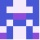Home
IT Knowledge
Inspiration
Languages
EN

# JavaScript - sum / subtract numbers in array with reduce()

0 points
Created by:Wesley
201

In this article, we would like to show you how to sum and subtract numbers with `reduce()` method in JavaScript.

## 1. Sum example

In below example, we execute `sum` function provided to the `reduce()` method that sums all the numbers in the array.

``````// ONLINE-RUNNER:browser;

const numbers = [1, 2, 3];
const sum = (accumulator, number) => accumulator + number;

console.log(numbers.reduce(sum)); //6``````

## 2. Subtract example

In below example, we execute `subtract` function provided to the `reduce()` method that subtracts all the numbers in the array from the `accumulator` (first item in the array).

``````// ONLINE-RUNNER:browser;

var numbers = [10, 1, 2];
const subtract = (accumulator, number) =>  accumulator - number;

console.log(numbers.reduce(subtract)); //7``````